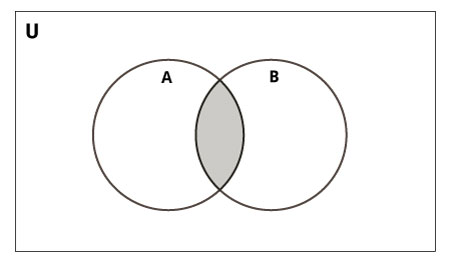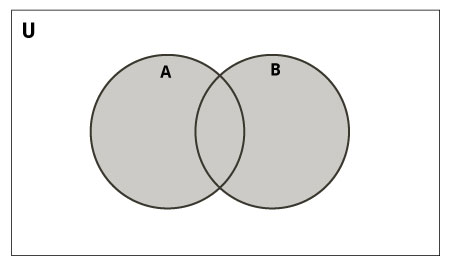# Find Union and Intersection of two linked lists in Python

In this tutorial, we learn to find the Union and Intersection of two linked lists in Python. The difference between the two sets is converted to a third set with elements common to both sets. The union between two sets is with all the elements from both sets in the third set.IntersectionUnion

The program creates two linked lists and finds its union and intersections.

### Algorithm

• Let’s get started by creating a node with instance data(integer type) and a next pointer.
• Then, Create a function prev_node, dup(duplicate), ins_end(insert at the end), display, and remove.
• The prev_node() function returns the previous node by taking a reference node as an argument.
• The ins_end function inserts a node at the end of the list.
• The display function will traverse the whole list and print the data(value) of each node.
• Remove function deletes it from the list by taking the node as an argument.
• The duplicate method returns a copy of the list.
• Define the function remove_dup that passes duplicate elements from the list as arguments.
• find_union function takes two linked lists as an argument and returns the union.
• So in the end, create a function find_intersec that two linked list as an argument and return the intersection of them.

### Python Program

Creating a class Node:

```class Node:
def __init__(self, data):
self.data = data
self.next = None```

Now create a class linked list:

```class LinkList:
def __init__(self):

def get_prev_node(self, ref_node):
while (current and current.next != ref_node):
current = current.next
return current

def duplicate(self):
while current:
node = Node(current.data)
copy.insert_at_end(node)
current = current.next
return copy

def insert_at_end(self, new_node):
else:
while current.next is not None:
current = current.next
current.next = new_node

def remove(self, node):
prev_node = self.get_prev_node(node)
if prev_node is None:
else:
prev_node.next = node.next

def display(self):
while current:
print(current.data, end = ' ')
current = current.next```

For intersections, we need to remove some remaining elements from the linked list, so we need to create a function to do this.

```def remove_duplicates(llist):
while current1:
current2 = current1.next
data = current1.data
while current2:
temp = current2
current2 = current2.next
if temp.data == data:
llist.remove(temp)
current1 = current1.next```

So, till now we had formed the basis for our program, now we have to create a function union and intersection.

```def find_union(llist1, llist2):
union = llist2.duplicate()
remove_duplicates(union)
return union
union = llist1.duplicate()
remove_duplicates(union)
return union

union = llist1.duplicate()
while last_node.next is not None:
last_node = last_node.next
llist2_copy = llist2.duplicate()
remove_duplicates(union)

return union

def find_intersection(llist1, llist2):

while current1:
data = current1.data
while current2:
if current2.data == data:
node = Node(data)
intersection.insert_at_end(node)
break
current2 = current2.next
current1 = current1.next
remove_duplicates(intersection)

return intersection```

We have created all the important classes and functions for our task, so now we have to call all those functions and classes from “main”.

```a_llist1 = LinkList()
data_list = input('Enter the elements of 1st linked list: ').split()
for data in data_list:
node = Node(int(data))
a_llist1.insert_at_end(node)
data_list = input('Enter the elements of 2nd linked list: ').split()
for data in data_list:
node = Node(int(data))
a_llist2.insert_at_end(node)

union = find_union(a_llist1, a_llist2)
intersection = find_intersection(a_llist1, a_llist2)

print('Their union will be: ')
union.display()
print()
print('Their intersection will be: ')
intersection.display()
print()```
```Enter the elements of 1st linked list: 1 2 4 5 4 5
Enter the elements of 2nd linked list: 6 5 1 3
Their union will be:
1 2 4 5 6 3
Their intersection will be:
1 5```